# CDS 2016 Maths Question Paper -1

1. If the roots of the equation lx2 + mx + m = 0 are in the ratio p : q, then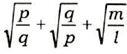is equal to
• 0
• 1
• 2
• 3
1. IF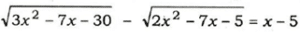has α and β  as its roots, then the value
of αβ is
• -15
• -5
• 0
• 5
1. If p/x+ q/y = m and q/x + p/y= n, then what is x/y equal to?
• np+mq/mp+nq
• np+mq/mp-nq
• np-mq/mp-nq
• np-mq/mp+nq
1. If a2 -by – cz – 0, ax – b2 + cz = 0 and ax + by – c2 =0, then the value of x/a+x+y/b+y+z/c+z will be
• a + b + c
• 3
• 1
• 0
1. If the equations x2-px + q= 0 and x2 +qx-p = 0 have a common root, then which one of the following is correct?
• p-q= 0
• p + q- 2 = 0
• p + q- l = 0
• p – q-1=0
1. If x = 21/3 + 2-1/3 then the value of 2x3 – 6x – 5 is equal to
• 0
• 1
• 2
• 3
1. The sum and difference of two expressions are 5x2-x-4 and x2 + 9x-10 respectively. The HCF of the two expressions will be
• (x+1)
• (x-l)
• (3x + 7)
• (2x – 3)
1. If (s – a) + (s – b) + (s – c) = s, then the value of (s-a)2 +(s-b)2 +(s-c)2 +s2/a2 + b2 + c2 will be
• 3
• 1
• 0
• -1
1. If the polynomial x6 + px5 + qx4– x2 – x – 3 is divisible by (x4 – 1), then the value of

p2 + q2 is

• 1
• 9
• 10
• 13
1. Let p and q be non-zero integers. Consider the polynomial

A(x) = x2 + px + q

It is given that (x – m) and (x – km) are simple factors of A(x), where m is a non-zero integer and k is a positive integer, k ≥2. Which one of the following is correct?

• (k + l)2p2 = kq
• (k + 1 )2q = kp2
• k2q = (k + l)p2
• k2 p2 = (k + l)2q
1. Let m be a non-zero integer and n be a positive integer. Let R be the remainder
obtained on dividing the polynomial xn +mn by (x-m). Then
• R is a non-zero even integer
• R is odd, if m is odd
• R = s2 for some integer s, if n is even
• R -t3 for some integer t, if 3 divides n
1. If 4x2y = 128 and 33x32y-9xy = 0, then the value of x + y can be equal to
• 7
• 5
• 3
• 1
1. If the linear factors of ax2 -(a2 + l)x + a are p and q, then p + q is equal to
• (x – l)(a + 1)
• (x + l)(a + 1)
• (x – l)(a- 1)
• (x + l)(a- 1)
1. If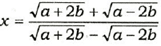then bx2 – ax + b is equal to (given that b≠ 0)
• 0
• 1
• ab
• 2ab
1. If a3 = 117 + b3 and a = 3 + b, then the value of a + b is ( given that a > 0 and
b > 0)
• 7
• 9
• 11
• 13
1. If the sum of the roots of ax2 + bx + c = 0 is equal to the sum of the squares of
their reciprocals, then which one of the following relations is correct?
• ab2 + bc2 = 2a2c
• ac2 + bc2=2b2a
• ab2 + bc2=a2c
• a2 + b2 + c2 = 1
1. Consider the following statements in respect of the expression sn=n(n+1)/2

where n is an integer :

1. There are exactly two values of n for which Sn = 861.
2. Sn = S-(n + 1) and hence for any integer m, we have two values of n for which Sn = m.

Which of the above statements is/are correct?

• 1 only
• 2 only
• Both 1 and 2
• Neither 1 nor 2
1. Consider the following statements in respect of two different non-zero integers p and q :
2. For (p + q) to be less than (p-q), q must be negative.
3. For (p + q) to be greater than (p-q), both p and q must be positive.

Which of the above statements is/are correct?

• 1 only
• 2 only
• Both 1 and 2
• Neither 1 nor 2
1. If a/b= b/c= c/d , then which of the following is/are correct?
2. b3 + c3 + d3/a3 +b3 + c3 =d/a
3. a2 + b2 + c2/b2 + c2 + d2 =a/d

Select the correct answer using the code given below.

• 1 only
• 2 only
• Both 1 and 2
• Neither 1 nor 2
1. 710-510 is divisible by
• 5
• 7
• 10
• 11
1. Let a two-digit number be k times the sum of its digits. If the number formed by interchanging the digits is m times the sum of the digits, then the value of m is
1. A man walking at 5 km/hour noticed that a 225 m long train coming in the opposite direction crossed him in seconds. The speed of the train is
• 75 km/hour
• 80 km/hour
• 85 km/hour
• 90 km/hour
1.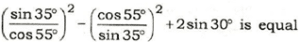to?
• -1
• 0
• 1
• 2
1. A cyclist moves non-stop from A to B, a distance of 14 km, at a certain average
If his average speed reduces by km per hour, he takes 20 minutes more to cover the same distance. The original average speed of the cyclist is
• 5 km/hour
• 6 km /hour
• 7 km/hour
• None of the above
1. If a sum of money at a certain rate of simple interest per year doubles in 5 years and at a different rate of simple interest per year becomes three times in 12 years, then the difference in the two rates of simple interest per year is
• 2%
• 3%
• 3 1/3 %
• 4 1/3%
1. Let ABC and A’B’C’ be two triangles in which AB>A’B’, BC> B’C’ and CA > C’A’. Let D, E and F be the mid- points of the sides BC, CA and AB respectively. Let D’, E’ and F’ be the midpoints of the sides B’C’, C’A’ and A’B’ respectively. Consider the following
statements :

Statement I :

AD > A’D’, BE > B’E’ and CF > C’F’ are always true.

Statement II :

AB2 + BC2+CA2/AD2 + BE2 + CF2=_ A’B’2+ B’C’2 + C’A’2/A’D’2+ B’E’2 + C’F’2

Which one of the following is correct in respect of the above statements?

• Both Statement I and Statement II are true and Statement II is the correct explanation of Statement I
• Both Statement I and Statement II are true but Statement II is not the correct explanation of Statement I
• Statement I is true but Statement II is false
• Statement I is false but Statement II is true
1. The annual incomes of two persons are in the ratio 9 : 7 and their expenses are
in the ratio 4:3. If each of them saves Rs.2,000 per year, what is the difference
in their annual incomes?
• 4,000
• 4,500
• 5,000
• 5,500
1. Let S be a set of first fourteen natural numbers. The possible number of pairs (a, b), where a, be S and a≠b such that ab leaves remainder 1 when divided by 15, is
• 3
• 5
• 6
• None of the above
1. A clock strikes once at 1 o’clock, twice at o’clock and thrice at 3 o’clock, and
so on. If it takes 8 seconds to strike at 5 o’clock, the time taken by it to strike
at 10 o’clock is
• 14 second
• 16 second
• 18 Second
• None of the above
1. In a circle of radius 2 units, a diameter AB intersects a chord of length 2 units
perpendicularly at P. If AP > BP, then AP is equal to
• (2+√5) units
• (2+√3) units
• (2+√2) units
• 3 units
1. Under what condition on p and q, one of the roots of the equation x2 + px + q = 0
is the square of the other?
• 1 + q + q2 = 3pq
• 1+ p + p2 = 3pq
• p3 + q + q2=3pq
• q3 + p + p2 = 3pq
1. What is the maximum value of m, if the number N = 90x42x324x55 is divisible by 3m?
• 8
• 7
• 6
• 5
1. A bike consumes 20 mL of petrol per kilometre, if it is driven at a speed in the
range of 25-50 km/hour and consumes mL of petrol per kilometre at any other speed. How much petrol is consumed by the bike in travelling a distance of 50 km, if the bike is driven at a speed of 40 km/hour for the first 10 km, at a speed of 60 km/hour
for the next 30 km and at a speed of 30 km/hour for the last 10 km?
• 1L
• 2 L
• 4 L
• 6 L
1. Consider the following statements :
2. Every natural number is a real number.
3. Every real number is a rational number.
4. Every integer is a real number.
5. Every rational number is a real number.

Which of the above statements are correct?

• 1, 2 and 3
• 1, 3 and 4
• 2 and 3 only
• 3 and 4 only
1. For what value of k is (x+ 1) a factor of x3 + kx2 – x + 2?
• 4
• 3
• 1
• -2
1. Consider the following statements :
2. There exists a positive real number m such that cosx = 2m + 1.
3. mn ≥ m + n for all m, n belonging to set of natural numbers.

Which of the above statements is/are correct?

• 1 only
• 2 only
• Both 1 and 2
• Neither 1 nor 2
1. The solution of the inequation 1 +I/x-1/x2 ≥ o is ( given that x ≠ 0)
• x > 0
• x<0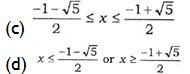1. Which of the points P( 5, -1), Q(3, -2) and R(l, 1) lie in the solution of the system of inequations x + y ≤ 4 and x-y ≥ 2?
• Q and R only
• P and R only
• P and Q only
• P, Q and R
1. A passenger train takes 1 hour less for a journey of 120 km, if its speed is increased by 10 km/hour from its usual speed. What is its usual speed?
• 50 km/hour
• 40 km/hour
• 35 km/hour
• 30 km/hour
1. The value of k, for which the system of equations 3x-ky-20 = 0 and 6x-10y+40=0 has no solution , is.
• 10
• 6
• 5
• 3
1. Consider the following in respect of natural numbers a, b and c :
2. LCM(ab, ac) = a LCM(b, c)
3. HCF(a£>, ac) = a HCF(b, c)
4. HCF(a, <b)<LCM(a, b)
5. HCF(a, b) divides LCM (a, b).
Which of the above are correct?
• 1 and 2 only
• 3 and 4 only
• 1, 2 and 4 only
• 1, 2, 3 and 4
1. There are three brothers. The sums of ages of two of them at a time are years, 6 years and 8 years. The age difference between the eldest and the youngest is
• 3 years
• 4 years
• 5 years
• 6 years
1. A person goes to a market between p.m. and 5 p.m. When he comes back, he finds that the hour hand and the minute hand of the clock have interchanged their positions. For how much time (approximately) was he out of his house?
• 38 minutes
• 58 minutes
• 57 Minutes
• 67 Minutes
1. In a gathering of 100 people, 70 of them can speak Hindi, 60 can speak English and 30 can speak French. Further, 30 of them can speak both Hindi and English, 20 can speak both Hindi and French. If x is the number of people who can speak both English and
French, then which one of the following is correct? (Assume that everyone can
speak at least one of the three languages.)
• 9 < x ≤ 30
• 0 ≤ x < 8
• x = 9
• x = 8
1. A cloth merchant buys cloth from a weaver and cheats him by using a scale which is 10 cm longer than a normal metre scale. He claims to sell cloth at the cost price to his customers, but while selling uses a scale which is cm shorter than a normal metre
What is his gain?
• 20%
• 21%
• 22 2/9%
• 23 1/3%
1. Two pipes A and B can fill a tank in 60 minutes and 75 minutes respectively. There is also an outlet C. If A, B and C are opened together, the tank is full in 50 minutes. How much time will be taken by C to empty the full tank?
• 100 minutes
• 110 minutes
• 120 minutes
• 125 minutes
1. In a race of 1000 m, A beats B by 100 m or 10 seconds. If they start a race of
1000 m simultaneously from the same point and if B gets injured after running
50 m less than half the race length and due to which his speed gets halved, then
by how much time will A beat B?
• 65 seconds
• 60 seconds
• 50 seconds
• 45 seconds
1. The salary of a person is increased by 10% of his original salary. But he received the same amount even after increment. What is the percentage of his salary he did not receive?
• 11%
• 10%
• (110/11)%
• (90/11)%
1. A truck moves along a circular path and describes 100 m when it has traced out
36° at the centre. The radius of the circle is equal to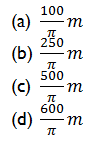1. Two poles are placed at P and Q on either side of a road such that the line joining
P and Q is perpendicular to the length of the road. A person moves x metre away
from P parallel to the road and places another pole at R. Then the person moves further x metre in the same direction and turns and moves a distance y metre away from the road perpendicularly, where he finds himself, Q and R on the same line. The distance between P and Q (i.e., the width of the road) in metre is
• X
• x/2
• y
• 2y
1. Suppose chords AB and CD of a circle intersect at a point P inside the circle.
Two right-angled triangles A’P’B’ and C’Q’D’ are formed as shown in the figures below such that A’P’ = AP, B’P’ = BP, C’Q’ = CP, D’Q’ = DP and ∠A’P’B’ = 90°= ∠C’Q’D’ :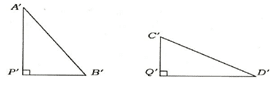Which of the following statements are not correct?

1. A’P’B’ and C’Q’D’ are similar triangles, but need not be congruent.
2. A’P’B’ and C’Q’D’ are congruent triangles.
3. A’P’B’ and C’Q’D’ are triangles of same area.
4. A’P’B’ and C’Q’D’ are triangles of same perimeter.

Select the correct answer using the code given below.

• 2 and 3 only
• 1 and 3 only
• 1, 2 and 4 only
• 1, 2, 3 and 4
1. Suppose ABC is a triangle with AB of unit length. D and E are the points lying on
AB and AC respectively such that BC and DE are parallel. If the area of triangle ABC is twice the area of triangle ADE, then the length of AD is
• ½ unit
• 1/3 unit
• 1/√2 unit
• 1/√3 unit
1. A rhombus is formed by joining midpoints of the sides of a rectangle in the suitable order. If the area of the rhombus is 2 square units, then the area of the rectangle is
• 2√2 square units
• 4 square units
• 4√2 square units
• 8 square units
1. If each interior angle of a regular polygon is 140°, then the number of vertices of
the polygon is equal to
• 10
• 9
• 8
• 7
1. Let the triangles ABC and DEF be such that ∠ABC = ∠DEF, ∠ACB = ∠DFE and
∠BAC = ∠EDF. Let L be the midpoint of BC and M be the midpoint of EF.
Consider the following statements :

Statement I :

Triangles ABL and DEM are similar.
Statement II :

Triangle ALC is congruent to triangle DMF even if AC ≠ DF.

Which one of the following is correct in respect of the above statements?

• Both Statement I and Statement II are true and Statement II is the correct explanation of Statement I
• Both Statement I and Statement II are true but Statement II is not the correct explanation of Statement I
• Statement I is true but Statement II is false
• Statement I is false but Statement II is true
1. The number of rounds that a wheel of diameter 7/11 metre will make in traversing 4 km will be
• 500
• 1000
• 1700
• 2000
1. The base of an isosceles triangle is 300 units and each of its equal sides is 170 units. Then the area of the triangle is
• 9600 square units
• 10000 square units
• 12000 square units
• None of the above
1. Four equal discs are placed such that each one touches two others. If the area of empty space enclosed by them is 150/847 square centimetre, then the radius of each disc is equal to
• 7/6 cm
• 5/6 cm
• 1 /2 cm
• 5/11 cm
1. ABC and DEF are similar triangles. If the ratio of side AB to side DE is (√2+1) :√3, then the ratio of area of triangle ABC to that of triangle DEF is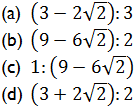1. A tangent is drawn from an external point O to a circle of radius 3 units at P such that OP = 4 units. If C is the centre of the circle, then the sine of the angle COP is
• 4/5
• 3/4
• 3/5
• ½
1. A square is inscribed in a right-angled triangle with legs p and q, and has a common right angle with the triangle. The diagonal of the square is given by
• Pq/p+2q
• Pq/2p+q
• √2pq/p+q
• 2pq/p+q
1.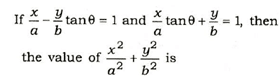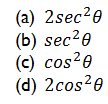1. Consider the following :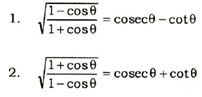Which of the above is/are identity/identities?

• 1 only
• 2 only
• Both 1 and 2
• Neither 1 nor 2
1. If p = cot θ+ tanθ and q = secθ -cosθ , then (p2q)2/3 -(q2p)2/3 is equal to
• 0
• 1
• 2
• 3
1. Two observers are stationed due north of a tower (of height x metre) at a distance
y metre from each other. The angles of elevation of the tower observed by them
are 30° and 45° respectively. Then x / y is equal to
•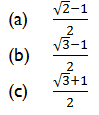• 1
1. If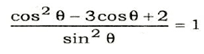where 0<θ<π/2  , then which of the following statements is/are correct?
2. There are two values of θ satisfying the above equation.
3. θ= 60° is satisfied by the above equation.

Select the correct answer using the code given below.

• 1 only
• 2 only
• Both 1 and 2
• Neither 1 nor 2
1. In a triangle ABC if A – B =π/2 , then C+ 2B is equal to
• 2π/3
• 3π/4
• π
• π/2
1. Which of the following is correct in respect of the equation 3-tan2 θ= a(1 – 3tan2 θ )? (Given that  is a real number.)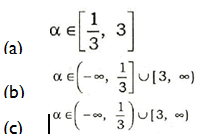• None of the above
1. There are five lines in a plane, no two of which are parallel. The maximum number of points in which they can intersect is
• 4
• 6
• 10
• None of the above
1. Consider a circle with centre at O and radius 7 cm. Let QR be a chord of length

cm and let P be the midpoint of QR. Let CD be another chord of this circle
passing through P such that ∠CPQ is acute. If M is the midpoint of CD and MP √24= cm, then which of the following statements are correct?

1. ∠QPD = 135°
2. If CP = m cm and PD = n cm, then m and n are the roots of the quadratic equation x2-10x+l = 0.
3. The ratio of the area of triangle OPR to the area of triangle OMP is 1: 2√2 .

Select the correct answer using the code given below.

• 1 and 2 only
• 2 and 3 only
• 1 and 3 only
• 1, 2 and 3
1. Let ABC be a triangle in which AB = AC. Let L be the locus of points X inside or on the triangle such that BX = CX. Which of the following statements are correct?
2. L is a straight line passing through A and in-centre of triangle ABC is on L.
3. L is a straight line passing through A and orthocentre of triangle ABC is a point on L.
4. L is a straight line passing through A and centroid of triangle ABC is a point on L.

Select the correct answer using the code given below.

• 1 and 2 only
• 2 and 3 only
• 1 and 3 only
• 1, 2 and 3
1. Consider a circle with centre at C. Let OP, OQ denote respectively the tangents to the circle drawn from a point O outside the circle. Let R be a point on OP and S be a point on OQ such that OR x SQ = OS x RP. Which of the following statements is/are correct?
2. If X is the circle with centre at O and radius OR, and Y is the circle with centre at O and radius OS, then X = Y.
3. ∠POC+ ∠QCO = 90°

Select the correct answer using the code given below.

• 1 only
• 2 only
• Both 1 and 2
• Neither 1 nor 2
1. If m and n are distinct natural numbers, then which of the following is/are integer / integers?
2. m/n+n/m
3. mn(m/n+n/m\(m2 +n2 )-1
4. mn/m2+n2

Select the correct answer using the code given below.

• 1 and 2
• 2 only
• 2 and 3
• 3 only
1. Outside a meeting room, Madhukar was told by a person that each meeting takes place after 13/4 hours. The last meeting has been over just 45 minutes ago and the next meeting will take place at 2 p.m. At what time did Madhukar receive this information?
• 10:20 a.m.
• 11:30 a.m.
• 11:40 a.m.
• 11:50 a.m.
1. The expenditure of a household for a certain month is Rs.20,000, out of which Rs.8,000 is spent on education, Rs.5,900 on food, Rs.2,800 on shopping and the rest on personal care. What percentage of expenditure is spent on personal care?
• 12%
• 5%
• 18%
• 8%
1. If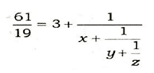where x, y and z are natural numbers, then what is z equal to?
• 1
• 2
• 3
• 4
1. A circular path is made from two concentric circular rings in such a way that the smaller ring when allowed to roll over the circumference of the bigger ring, it takes three full revolutions. If the area of the pathway is equal to n times the area of the smaller ring, then n is equal to
• 4
• 6
• 8
• 10
1. Consider the following statements :
2. If n ≥ 3 and m ≥ 3 are distinct positive integers, then the sum of the exterior angles of a regular polygon of m sides is different from the sum of the exterior angles of a regular polygon of n sides.
3. Let m, n be integers such that m > n ≥ 3. Then the sum of the interior angles of a regular polygon of m sides is greater than the sum of the interior angles of a
regular polygon of n sides, and their sum is (m + n)π/2 .

Which of the above statements is/are correct?

• 1 only
• 2 only
• Both 1 and 2
• Neither 1 nor 2
1. Consider the following statements :
2. There exists a regular polygon whose exterior angle is 70°.
3. Let n ≥ 5. Then the exterior angle of any regular polygon of n sides is acute.

Which of the above statements is/are correct?

• 1 only
• 2 only
• Both 1 and 2
• Neither 1 nor 2
1. In a triangle PQR, point X is on PQ and point Y is on PR such that XP = 1-5 units, XQ = 6 units, PY = 2 units and YR = 8 units. Which of the following are correct?
2. QR=5XY
3. QR is parallel to XY.
4. Triangle PYX is similar to triangle PRQ.

Select the correct answer using the code given below.

• 1 and 2 only
• 2 and 3 only
• I and 3 only
• 1, 2 and 3
1. If a transversal intersects four parallel straight lines, then the number of distinct values of the angles formed will be
• 2
• 4
• 8
• 16
1. A person travels 7 km eastwards and then turns right and travels 3 km and further turns right and travels 13 km. What is the shortest distance of the present position of the person from his starting point?
• 6 km
• 3√5 km
• 7 km
• 4√5 km
1. ABC is a triangle in which D is the midpoint of BC and E is the midpoint of AD. Which of the following statements is/are correct?
2. The area of triangle ABC is equal to four times the area of triangle BED.
3. The area of triangle ADC is twice the area of triangle BED.

Select the correct answer using the code given below.

• 1 only
• 2 only
• Both 1 and 2
• Neither 1 nor 2
1. A circle of 3 m radius is divided into three areas by semicircles of radii 1 m and 2 m as shown in the figure above. The ratio of the three areas A, B and C will be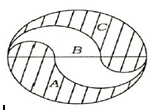• 2:3:2
• 1:1:1
• 4:3:4
• 1:2:1
1.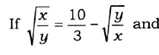x-y – 8, then the value of xy is equal to
• 36
• 24
• 16
• 9
1. ABC is a triangle right angled at C as shown in the figure above. Which one of the following is correct?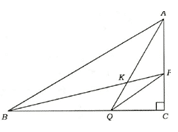• AQ2 + AB2 = BP2 + PQ2
• AQ2 + PQ2 = AB2 + BP2
• AQ2 + BP2 = AB2 + PQ2
• AQ2 + AP2 =BK2 + KQ2
1. In the figure given above, AD = CD = BC. What is the value of ∠CDB?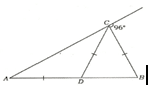• 32°
• 64°
• 78°
• Cannot be determined due to insufficient data
1. ABC is an equilateral triangle and X, Y and Z are the points on BC, CA and AB
respectively such that BX = CY = AZ. Which of the following is/are correct?
2. XYZ is an equilateral triangle.
3. Triangle XYZ is similar to triangle ABC.

Select the correct answer using the code given below.

• 1 only
• 2 only
• Both 1 and 2
• Neither 1 nor 2
1. In the figure given above, p and q are parallel lines. What are the values of the
angles x, y and z?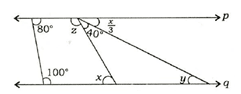• x = 80°, y = 40°,z=100°
• x = 80°, y = 50°,z=105°
• x = 70°, y = 40°,z = 110°
• x = 60°, y = 20°,z=120°
1. The linear inequations, for which the shaded area in the figure given above is
the solution set, are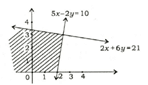• 2x + 6y ≤ 2l, 5x-2y ≤10
• 2x + 6y ≤ 2l, bx-2y ≥10
• 2x + 6y ≥ 21, 5x – 2y ≤ 10
• 2x + 6y ≥ 21, 5x-2y ≥10
1. The election result in which six parties contested was depicted by a pie chart. Party A had an angle 135° on this pie chart. If it secured 21960 votes, how many valid votes in total were cast?
• 51240
• 58560
• 78320
• 87840
1. The mean and median of 5 observations are 9 and 8 respectively. If 1 is subtracted from each observation, then the new mean and the new median will respectively be
• 8 and 7
• 9 and 7
• 8 and 9
• Cannot be determined due to insufficient data
1. The age distribution of 40 children is as follows :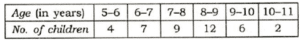Consider the following statements in respect of the above frequency distribution :

1. The median of the age distribution is 7 years.
2. 70% of the children are in the age group 6-9 years.
3. The modal age of the children is 8 years.

Which of the above statement are correct?

• 1 and 2 only
• 2 and 3 only
• 1 and 3 only
• 1,2,and 3
1. Suppose xi i for 0 ≤ i ≤ 10, where > 1.Which one of the following is correct?
• AM < Median
• GM < Median
• GM = Median
• AM = Median
1. Suppose Xi = 1/i for i = 1, 2, 3,…, 11. Which one of the following is not correct?
• AM >1/6
• GM >1/6
• HM > 1/6
• Median = HM
1. The value of 1/5log10 3125 -41og10 2 + log10 32 is
• 0
• 1
• 2
• 3
1. If tanθ + cot θ =4/√3 =, where 0 < θ  <π/2 , then sinθ +cosθ  is equal to
• 1
• √3-1/2
• √3+1/2
• √2
1. The value of the expression (243 + 647)2 + (243 – 647)2/243×243 + 647×647
is equal to
• 0
• 1
• 2
• 3
1. If a and b are negative real numbers and c is a positive real number, then which
of the following is/are correct?
2. a-b < a-c
3. if a<b, than a/c,b/c
4. 1/b<1/c

Select the correct answer given below.

• 1
• 2 only
• 3Only
• 2 and 3
1. AD is the diameter of a circle with area 707 m2 and AB = BC= CD as shown in
the figure above. All curves inside the circle are semicircles with their diameters on AD. What is the cost of levelling the shaded region at the rate of Rs.63 per square metre?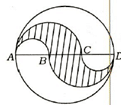• 29,700
• 22,400
• 14,847
• None of the above